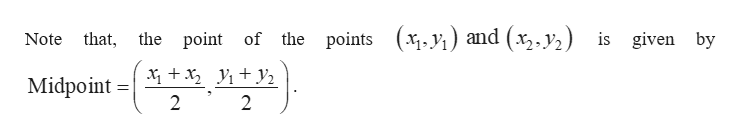# Find the coordinates of the other endpoint of the​ segment, given its midpoint and one endpoint.​ (Hint: Let​ (x,y) be the unknown endpoint. Apply the midpoint​ formula, and solve the two equations for x and​ y.) midpoint​(15​,7​),endpoint​(14​,16​)

Question
9 views
Find the coordinates of the other endpoint of the​ segment, given its midpoint and one endpoint.​ (Hint: Let​ (x,y) be the unknown endpoint. Apply the midpoint​ formula, and solve the two equations for x and​ y.)

midpoint
​(15​,7​),
endpoint
​(14​,16​)
check_circle

Step 1

Consider the one end point and midpoint as (14, 16) and (15, 7).

Let the ...help_outlineImage TranscriptioncloseNote that, the point of the points (x,y) and (x2. y2) is given by Midpoint 2 2 fullscreen

### Want to see the full answer?

See Solution

#### Want to see this answer and more?

Solutions are written by subject experts who are available 24/7. Questions are typically answered within 1 hour.*

See Solution
*Response times may vary by subject and question.
Tagged in

### Other